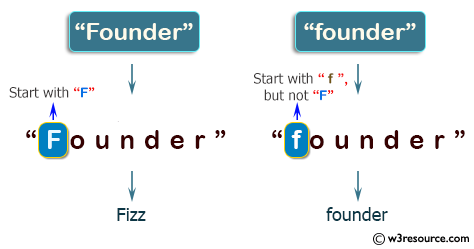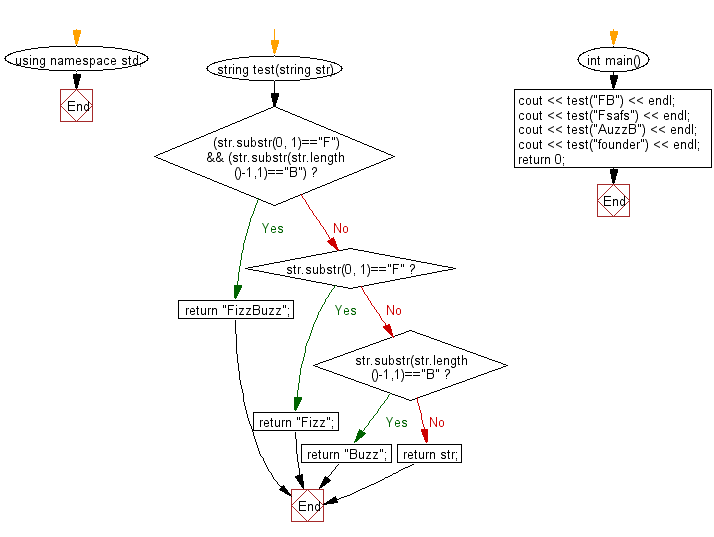﻿ C++ : Check if a given string starts with F or ends with B# C++ Exercises: Check whether a given string starts with "F" or ends with "B"

## C++ Basic Algorithm: Exercise-43 with Solution

Write a C++ program to check whether a given string starts with "F" or ends with "B". If the string starts with "F" return "Fizz" and return "Buzz" if it ends with "B" If the string starts with "F" and ends with "B" return "FizzBuzz". In other cases return the original string.

Sample Solution:

C++ Code :

``````#include <iostream>

using namespace std;

string test(string str)
{
if ((str.substr(0, 1)=="F") && (str.substr(str.length()-1,1)=="B"))
{
return "FizzBuzz";
}
else if (str.substr(0, 1)=="F")
{
return "Fizz";
}
else if (str.substr(str.length()-1,1)=="B")
{
return "Buzz";
}
else
{
return str;
}
}

int main()
{
cout << test("FB") << endl;
cout << test("Fsafs") << endl;
cout << test("AuzzB") << endl;
cout << test("founder") << endl;
return 0;
}
``````

Sample Output:

```FizzBuzz
Fizz
Buzz
Founder
```

Pictorial Presentation:Flowchart:C++ Code Editor:

Contribute your code and comments through Disqus.

What is the difficulty level of this exercise?

﻿